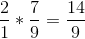## Example Questions

### Example Question #1 : How To Multiply Complex Fractions

What is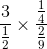?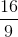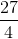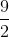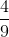Explanation:

Simplify both sides first.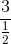simplifies to 6.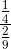simplifies to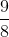. Finally 6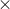=.

### Example Question #2 : How To Multiply Complex Fractions

What is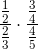equal to?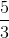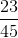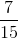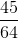Explanation:

When multiplying fractions, we can simply multiply the numerators and then multiply the denominators. Therefore,is equal to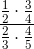We then do the same thing again, giving us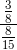.

Now we must find the least common denominator, which is.

We multiply the top by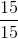and the bottom by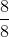. After we do this we can multiply our numerator by the reciprocal of the denominator.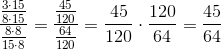.

### Example Question #3 : How To Multiply Complex Fractions

Simplify: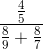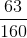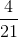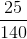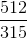Explanation:

Begin by simplifying the denominator: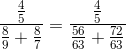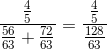Then, you perform the division by multiplying the numerator by the reciprocal of the denominator: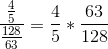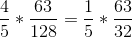Finally, multiply everything: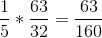### Example Question #4 : How To Multiply Complex Fractions

Simplify: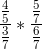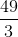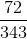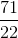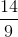Explanation:

Generally, when you multiply fractions, it is a very easy affair. This does not change for complex fractions like this. You can begin by simply multiplying the numerators and denominators directly. Thus, you know: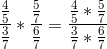Now, simplify this to: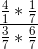or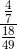Now, remember that when you divide fractions, you multiply the numerator by the reciprocal of the denominator: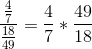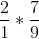, which is easy to finish: